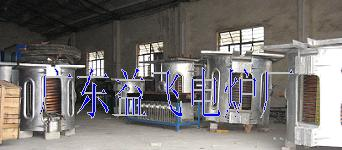## How is the current of induction furnace transformed into heat energy?

Time：2021-02-14   Nums：1692

Joule Lenz law reveals the law of transforming electric energy into heat energy, and also lays the theoretical foundation of induction heating and the working principle of induction furnace.

1) The meaning of Joule Lenz current heat effect law

When the current passes through the conductor, the heat emitted by overcoming the obstruction of the conductor is proportional to the square of the current, the resistance of the conductor and the time of power on.

The expression of current thermal effect is as follows

Q=IRt

In the formula, Q --- heat converted from electric energy (J)

I -- current through conductor (a)

T --- power on time (s)

R --- effective resistance of conductor

2) Thermal effect of induced current

When the induced current flows in the loop, the free electrons must consume part of the energy to do work in order to overcome various resistances, so that the electric energy overcoming the conductor resistance can be converted into heat energy. The heat energy produced by the induction current is the only heat source in the induction furnace.

The working principle of induction melting furnace is the system conversion process of electric energy, magnetic energy, electric energy, thermal energy, metal heating and melting.Yiphee Electric Furnace Co.,Ltd specializes in producing induction smelting furnace.Tel(WhatsApp):+86-13450756789, yiphee@yiphee.cn